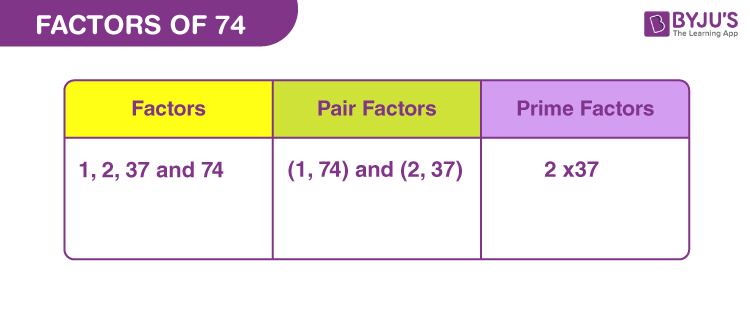# Factors of 74

Factors of 74 are the natural numbers that uniformly divide the actual number. 74 is an even number, therefore, it is divisible by 2 (by divisibility rules). Thus, the number 2 divides the original number into two equal parts. The factors are also called the divisors of 74.

If the product of two whole numbers is equal to 74, then such numbers are the required factors. Since, 74 is a composite number, hence it should have more than two factors. In this article, we will discuss to find the factors of 74 along with pair factors and prime factors.## How to Find the Factors of 74?

74 is divisible by its factors. Therefore, we can divide 74 by different natural numbers, to check by which number, it is completely divisible. Also, we can check by multiplying the factors to get the original number.

 Division Method Multiplication Method 74 ÷ 1 = 74 1 x 74 = 74 74 ÷ 2 = 37 2 x 37 = 74 74 ÷ 37 = 2 37 x 2 = 74 74 ÷ 74 = 1 74 x 1 = 74

Therefore, the factors of 74 are 1, 2, 37 and 74.

## Pair Factors of 74

When we multiply factors in pairs and the product is equal to 74, then they are said to be pair factors.

• 1 × 74 = 74
• 2 x 37 = 74

Therefore, the pair factors of 74 are (1, 74) and (2, 37).

Multiplication of two negative numbers will always result in a positive number. Therefore,

• -1 × -74 = 74
• -2 × -37 = 74

Therefore, the negative pair factors are (-1, -74) and (-2, -37).

## Prime Factorisation of 74

To find the prime factors of 74, we can simply use the prime factorisation method. These prime factors are also the uniform divisors of 74.

According to prime factorisation, we divide the original number by the list of prime numbers, starting from the smallest prime number, till we get the quotient equal to 1. Hence,

 Dividing 74 by smallest prime number 74 ÷ 2 = 37 37 is a prime number and is divisible by itself 37 ÷ 37 = 1

Therefore, the prime factors of 74 are 2 and 37.

 Prime factorisation of 74 = 2 x 37 Exponential form = 21 x 371

Also check: Prime Numbers

## Solved Examples

Q.1: Sarita bought 74 cookies. She wants to put these cookies equally in two jars. How can she do so?

Solution: Number of cookies = 74

Number of jars = 2

Therefore, she can put 37 cookies in each of the jars.

Q.2: What is the sum of all the factors of 74?

Solution: The factors of 74 are 1, 2, 37 and 74.

Sum = 1 + 2 + 37 + 74 = 114

Therefore, the required sum is 114.

Q.3: What are the common factors of 74 and 84?

Solution: We have to list the factors of 74 and 84.

74 → 1, 2, 37, 74

84 → 1, 2, 3, 4, 6, 7, 12, 14, 21, 28, 42, 84

Therefore, the common factors of 74 and 84 are 1 and 2.

Q.4: What is the GCF of 74 and 92?

Solution: The factors of the respective numbers are:

74 → 1, 2, 37, 74

92 → 1, 2, 4, 23, 46, 92

Therefore, the GCF of 74 and 92 is 2.

### Practice Questions

1. What is the average of factors of 74?
2. How many factors are there for number 74?
3. What is the common factor of 74 and 75?
4. Find the GCF of 74 and 90.

## Frequently Asked Questions on Factors of 74

### What are the factors of 74?

The factors of 74 are 1, 2, 37 and 74.

### What are the multiples of 74?

The first ten multiples of 74 are 74, 148, 222, 296, 370, 444, 518, 592, 666 and 740.

### Is 74 a prime number or composite number?

74 is a composite number because it has more than two factors.

### What are the prime factors of 74?

Answer: The prime factors of 74 are 2 and 37. Also, the product of both prime factors is equal to 74.

2 x 37 = 74

### What are the pair factors of 74?

The pair factors of 74 are (1, 74) and (2, 37)

### What is the GCF of 74 and 100?

The GCF (greatest common factor) of 74 and 100 is 2.